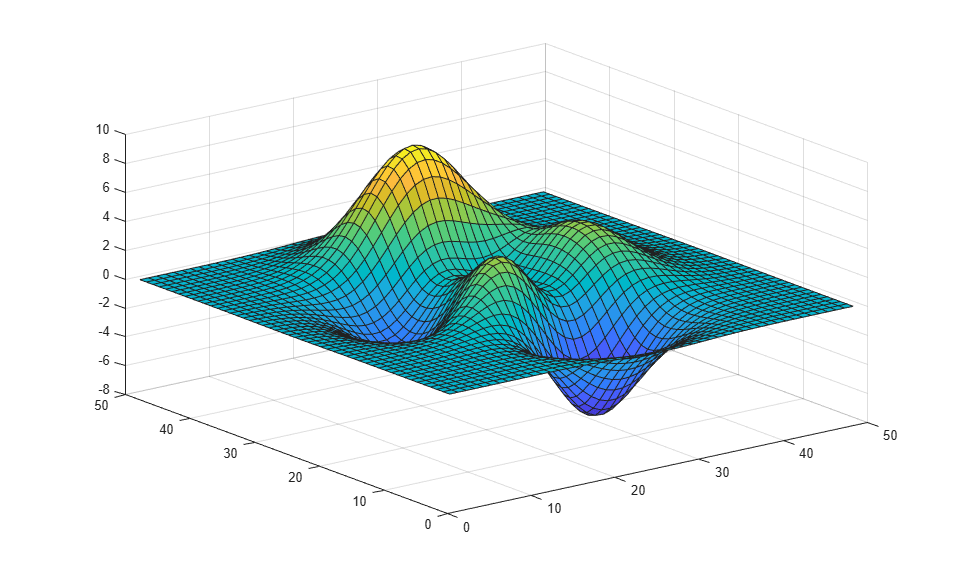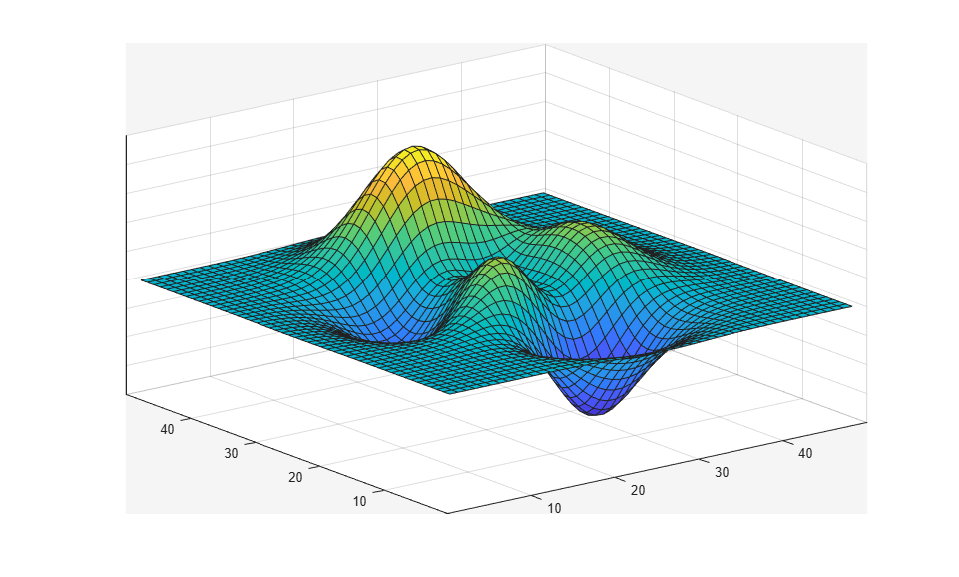# frame2im

## 语法

``RGB = frame2im(F)``
``[X,map] = frame2im(F)``

## 说明

``RGB = frame2im(F)` 从单个影片帧 `F` 返回真彩色 (RGB) 图像。`
``[X,map] = frame2im(F)` 从单个影片帧 `F` 返回索引图像数据 `X` 和关联的颜色图 `map`。`

## 示例

```surf(peaks) ``````F = getframe ```
```F = struct with fields: cdata: [357x452x3 uint8] colormap: [] ```

```RGB = frame2im(F); ```

```figure imshow(RGB) ```## 输入参数

• `cdata` - 存储为 `uint8` 值数组的图像数据。

• `colormap` - 颜色图。如果影片帧包含一个真彩色 (RGB) 图像，则此字段为空 (`[]`)。